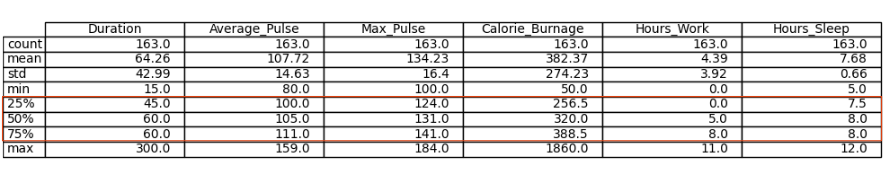# Data Science - Statistics Percentiles

## 25%, 50% and 75% - Percentiles

Percentiles are used in statistics to give you a number that describes the value that a given percent of the values are lower than.Let us try to explain it by some examples, using Average_Pulse.

• The 25% percentile of Average_Pulse means that 25% of all of the training sessions have an average pulse of 100 beats per minute or lower. If we flip the statement, it means that 75% of all of the training sessions have an average pulse of 100 beats per minute or higher
• The 75% percentile of Average_Pulse means that 75% of all the training session have an average pulse of 111 or lower. If we flip the statement, it means that 25% of all of the training sessions have an average pulse of 111 beats per minute or higher

### Task: Find the 10% percentile for Max_Pulse

The following example shows how to do it in Python:

### Example

import numpy as np

Max_Pulse= full_health_data["Max_Pulse"]
percentile10 = np.percentile(Max_Pulse, 10)
print(percentile10)
Try it Yourself »
• Max_Pulse = full_health_data["Max_Pulse"] - Isolate the variable Max_Pulse from the full health data set.
• np.percentile() is used to define that we want the 10% percentile from Max_Pulse.

The 10% percentile of Max_Pulse is 120. This means that 10% of all the training sessions have a Max_Pulse of 120 or lower.

W3Schools is optimized for learning and training. Examples might be simplified to improve reading and learning. Tutorials, references, and examples are constantly reviewed to avoid errors, but we cannot warrant full correctness of all content. While using W3Schools, you agree to have read and accepted our terms of use, cookie and privacy policy.